## 10.1 Rotational Variables

### Learning Objectives

By the end of this section, you will be able to:

• Describe the physical meaning of rotational variables as applied to fixed-axis rotation
• Explain how angular velocity is related to tangential speed
• Calculate the instantaneous angular velocity given the angular position function
• Find the angular velocity and angular acceleration in a rotating system
• Calculate the average angular acceleration when the angular velocity is changing
• Calculate the instantaneous angular acceleration given the angular velocity function

So far in this text, we have mainly studied translational motion, including the variables that describe it: displacement, velocity, and acceleration. Now we expand our description of motion to rotation—specifically, rotational motion about a fixed axis. We will find that rotational motion is described by a set of related variables similar to those we used in translational motion.

### Angular Velocity

Uniform circular motion (discussed previously in Motion in Two and Three Dimensions) is motion in a circle at constant speed. Although this is the simplest case of rotational motion, it is very useful for many situations, and we use it here to introduce rotational variables.

In (Figure), we show a particle moving in a circle. The coordinate system is fixed and serves as a frame of reference to define the particle’s position. Its position vector from the origin of the circle to the particle sweeps out the angle $\theta$, which increases in the counterclockwise direction as the particle moves along its circular path. The angle $\theta$ is called the angular position of the particle. As the particle moves in its circular path, it also traces an arc length s.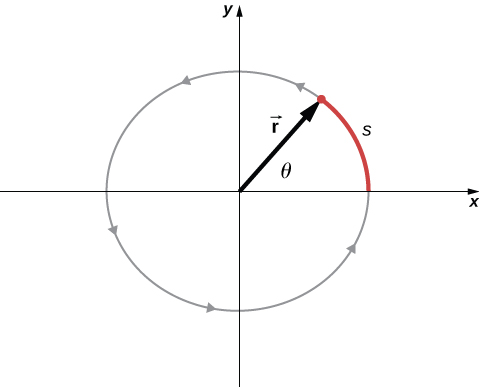Figure 10.2 A particle follows a circular path. As it moves counterclockwise, it sweeps out a positive angle $\theta$ with respect to the x-axis and traces out an arc length s.

The angle is related to the radius of the circle and the arc length by

$\theta =\frac{s}{r}.$

The angle $\theta$, the angular position of the particle along its path, has units of radians (rad). There are $2\pi$ radians in $360\text{°}.$ Note that the radian measure is a ratio of length measurements, and therefore is a dimensionless quantity. As the particle moves along its circular path, its angular position changes and it undergoes angular displacements $\text{Δ}\theta .$

We can assign vectors to the quantities in (Figure). The angle $\overset{\to }{\theta }$ is a vector out of the page in (Figure). The angular position vector $\overset{\to }{r}$ and the arc length $\overset{\to }{s}$ both lie in the plane of the page. These three vectors are related to each other by

$\overset{\to }{s}=\overset{\to }{\theta }\,×\,\overset{\to }{r}.$

That is, the arc length is the cross product of the angle vector and the position vector, as shown in (Figure).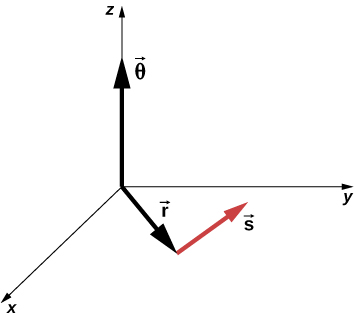Figure 10.3 The angle vector points along the z-axis and the position vector and arc length vector both lie in the xy-plane. We see that $\overset{\to }{s}=\overset{\to }{\theta }\,×\,\overset{\to }{r}$. All three vectors are perpendicular to each other.

The magnitude of the angular velocity, denoted by $\omega$, is the time rate of change of the angle $\theta$ as the particle moves in its circular path. The instantaneous angular velocity is defined as the limit in which $\text{Δ}t\to 0$ in the average angular velocity $\overset{–}{\omega }=\frac{\text{Δ}\theta }{\text{Δ}t}$:

$\omega =\underset{\text{Δ}t\to 0}{\text{lim}}\frac{\text{Δ}\theta }{\text{Δ}t}=\frac{d\theta }{dt},$

where $\theta$ is the angle of rotation ((Figure)). The units of angular velocity are radians per second (rad/s). Angular velocity can also be referred to as the rotation rate in radians per second. In many situations, we are given the rotation rate in revolutions/s or cycles/s. To find the angular velocity, we must multiply revolutions/s by $2\pi$, since there are $2\pi$ radians in one complete revolution. Since the direction of a positive angle in a circle is counterclockwise, we take counterclockwise rotations as being positive and clockwise rotations as negative.

We can see how angular velocity is related to the tangential speed of the particle by differentiating (Figure) with respect to time. We rewrite (Figure) as

$s=r\theta .$

Taking the derivative with respect to time and noting that the radius r is a constant, we have

$\frac{ds}{dt}=\frac{d}{dt}(r\theta )=\theta \frac{dr}{dt}+r\frac{d\theta }{dt}=r\frac{d\theta }{dt}$

where $\theta \frac{dr}{dt}=0$. Here $\frac{ds}{dt}$ is just the tangential speed ${v}_{\text{t}}$ of the particle in (Figure). Thus, by using (Figure), we arrive at

${v}_{\text{t}}=r\omega .$

That is, the tangential speed of the particle is its angular velocity times the radius of the circle. From (Figure), we see that the tangential speed of the particle increases with its distance from the axis of rotation for a constant angular velocity. This effect is shown in (Figure). Two particles are placed at different radii on a rotating disk with a constant angular velocity. As the disk rotates, the tangential speed increases linearly with the radius from the axis of rotation. In (Figure), we see that ${v}_{1}={r}_{1}{\omega }_{1}$ and ${v}_{2}={r}_{2}{\omega }_{2}$. But the disk has a constant angular velocity, so ${\omega }_{1}={\omega }_{2}$. This means $\frac{{v}_{1}}{{r}_{1}}=\frac{{v}_{2}}{{r}_{2}}$ or ${v}_{2}=(\frac{{r}_{2}}{{r}_{1}}){v}_{1}$. Thus, since ${r}_{2}>{r}_{1}$, ${v}_{2}>{v}_{1}$.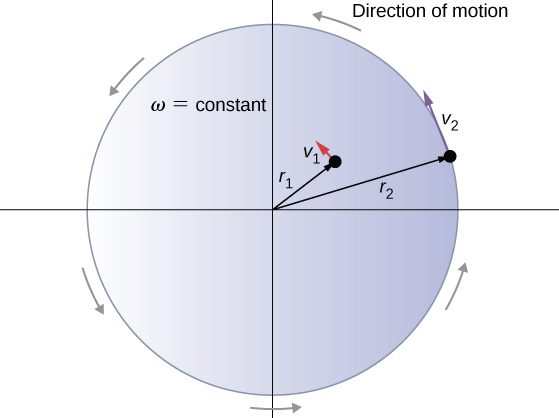Figure 10.4 Two particles on a rotating disk have different tangential speeds, depending on their distance to the axis of rotation.

Up until now, we have discussed the magnitude of the angular velocity $\omega =d\theta \text{/}dt,$ which is a scalar quantity—the change in angular position with respect to time. The vector $\overset{\to }{\omega }$ is the vector associated with the angular velocity and points along the axis of rotation. This is useful because when a rigid body is rotating, we want to know both the axis of rotation and the direction that the body is rotating about the axis, clockwise or counterclockwise. The angular velocity $\overset{\to }{\omega }$ gives us this information. The angular velocity $\overset{\to }{\omega }$ has a direction determined by what is called the right-hand rule. The right-hand rule is such that if the fingers of your right hand wrap counterclockwise from the x-axis (the direction in which $\theta$ increases) toward the y-axis, your thumb points in the direction of the positive z-axis ((Figure)). An angular velocity $\overset{\to }{\omega }$ that points along the positive z-axis therefore corresponds to a counterclockwise rotation, whereas an angular velocity $\overset{\to }{\omega }$ that points along the negative z-axis corresponds to a clockwise rotation.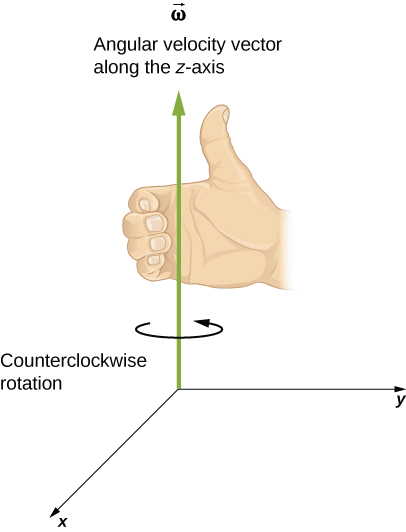Figure 10.5 For counterclockwise rotation in the coordinate system shown, the angular velocity points in the positive z-direction by the right-hand-rule.

We can verify the right-hand-rule using the vector expression for the arc length $\overset{\to }{s}=\overset{\to }{\theta }\,×\,\overset{\to }{r}$, (Figure). If we differentiate this equation with respect to time, we find

$\frac{d\overset{\to }{s}}{dt}=\frac{d}{dt}(\overset{\to }{\theta }\,×\,\overset{\to }{r})=(\frac{d\overset{\to }{\theta }}{dt}\,×\,\overset{\to }{r})+(\overset{\to }{\theta }\,×\,\frac{d\overset{\to }{r}}{dt})=\frac{d\overset{\to }{\theta }}{dt}\,×\,\overset{\to }{r}.$

Since $\overset{\to }{r}$ is constant, the term $\overset{\to }{\theta }\,×\,\frac{d\overset{\to }{r}}{dt}=0$. Since $\overset{\to }{v}=\frac{d\overset{\to }{s}}{dt}$ is the tangential velocity and $\overset{\to }{\omega }=\frac{d\overset{\to }{\theta }}{dt}$ is the angular velocity, we have

$\overset{\to }{v}=\overset{\to }{\omega }\,×\,\overset{\to }{r}.$

That is, the tangential velocity is the cross product of the angular velocity and the position vector, as shown in (Figure). From part (a) of this figure, we see that with the angular velocity in the positive z-direction, the rotation in the xy-plane is counterclockwise. In part (b), the angular velocity is in the negative z-direction, giving a clockwise rotation in the xy-plane.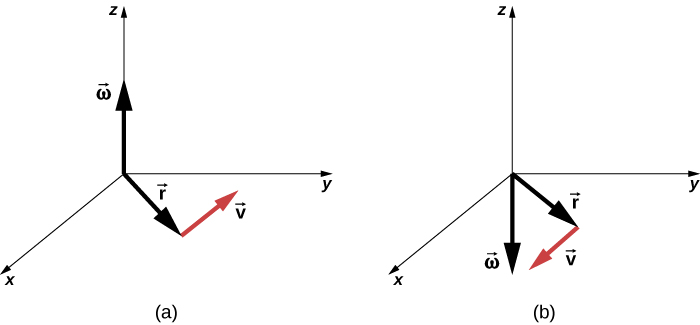Figure 10.6 The vectors shown are the angular velocity, position, and tangential velocity. (a) The angular velocity points in the positive z-direction, giving a counterclockwise rotation in the xy-plane. (b) The angular velocity points in the negative z-direction, giving a clockwise rotation.

### Example

#### Rotation of a Flywheel

A flywheel rotates such that it sweeps out an angle at the rate of $\theta =\omega t=(45.0\,\text{rad}\text{/}\text{s)}t$ radians. The wheel rotates counterclockwise when viewed in the plane of the page. (a) What is the angular velocity of the flywheel? (b) What direction is the angular velocity? (c) How many radians does the flywheel rotate through in 30 s? (d) What is the tangential speed of a point on the flywheel 10 cm from the axis of rotation?

#### Strategy

The functional form of the angular position of the flywheel is given in the problem as $\theta (t)=\omega t$, so by taking the derivative with respect to time, we can find the angular velocity. We use the right-hand rule to find the angular velocity. To find the angular displacement of the flywheel during 30 s, we seek the angular displacement $\text{Δ}\theta$, where the change in angular position is between 0 and 30 s. To find the tangential speed of a point at a distance from the axis of rotation, we multiply its distance times the angular velocity of the flywheel.

#### Solution

1. $\omega =\frac{d\theta }{dt}=45\,\text{rad}\text{/}\text{s}$. We see that the angular velocity is a constant.
2. By the right-hand rule, we curl the fingers in the direction of rotation, which is counterclockwise in the plane of the page, and the thumb points in the direction of the angular velocity, which is out of the page.
3. $\text{Δ}\theta =\theta (30\,\text{s})-\theta (0\,\text{s})=45.0(30.0\,\text{s})-45.0(0\,\text{s})=1350.0\,\text{rad}$.
4. ${v}_{\text{t}}=r\omega =(0.1\,\text{m})(45.0\,\text{rad}\text{/}\text{s})=4.5\,\text{m/s}$.

#### Significance

In 30 s, the flywheel has rotated through quite a number of revolutions, about 215 if we divide the angular displacement by $2\pi$. A massive flywheel can be used to store energy in this way, if the losses due to friction are minimal. Recent research has considered superconducting bearings on which the flywheel rests, with zero energy loss due to friction.

### Angular Acceleration

We have just discussed angular velocity for uniform circular motion, but not all motion is uniform. Envision an ice skater spinning with his arms outstretched—when he pulls his arms inward, his angular velocity increases. Or think about a computer’s hard disk slowing to a halt as the angular velocity decreases. We will explore these situations later, but we can already see a need to define an angular acceleration for describing situations where $\omega$ changes. The faster the change in $\omega$, the greater the angular acceleration. We define the instantaneous angular acceleration $\alpha$ as the derivative of angular velocity with respect to time:

$\alpha =\underset{\text{Δ}t\to 0}{\text{lim}}\frac{\text{Δ}\omega }{\text{Δ}t}=\frac{d\omega }{dt}=\frac{{d}^{2}\theta }{d{t}^{2}},$

where we have taken the limit of the average angular acceleration, $\overset{–}{\alpha }=\frac{\text{Δ}\omega }{\text{Δ}t}$ as $\text{Δ}t\to 0$.

The units of angular acceleration are (rad/s)/s, or ${\text{rad/s}}^{2}$.

In the same way as we defined the vector associated with angular velocity $\overset{\to }{\omega }$, we can define $\overset{\to }{\alpha }$, the vector associated with angular acceleration ((Figure)). If the angular velocity is along the positive z-axis, as in (Figure), and $\frac{d\omega }{dt}$ is positive, then the angular acceleration $\overset{\to }{\alpha }$ is positive and points along the $+z\text{-}$ axis. Similarly, if the angular velocity $\overset{\to }{\omega }$ is along the positive z-axis and $\frac{d\omega }{dt}$ is negative, then the angular acceleration is negative and points along the $+z-$ axis.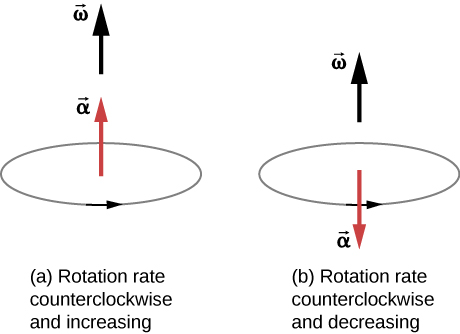Figure 10.7 The rotation is counterclockwise in both (a) and (b) with the angular velocity in the same direction. (a) The angular acceleration is in the same direction as the angular velocity, which increases the rotation rate. (b) The angular acceleration is in the opposite direction to the angular velocity, which decreases the rotation rate.

We can express the tangential acceleration vector as a cross product of the angular acceleration and the position vector. This expression can be found by taking the time derivative of $\overset{\to }{v}=\overset{\to }{\omega }\,×\,\overset{\to }{r}$ and is left as an exercise:

$\overset{\to }{\mathbf{\text{a}}}=\overset{\to }{\mathbf{\text{α}}}\,×\,\overset{\to }{\mathbf{\text{r}}}.$

The vector relationships for the angular acceleration and tangential acceleration are shown in (Figure).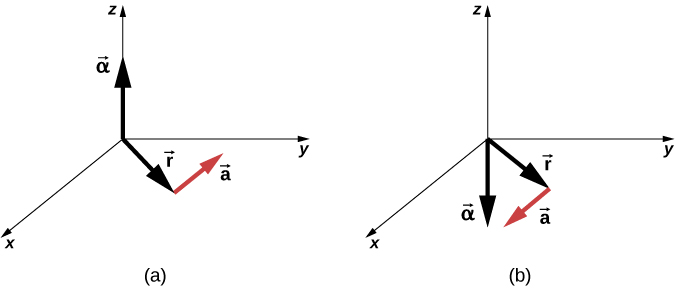Figure 10.8 (a) The angular acceleration is the positive z-direction and produces a tangential acceleration in a counterclockwise sense. (b) The angular acceleration is in the negative z-direction and produces a tangential acceleration in the clockwise sense.

We can relate the tangential acceleration of a point on a rotating body at a distance from the axis of rotation in the same way that we related the tangential speed to the angular velocity. If we differentiate (Figure) with respect to time, noting that the radius r is constant, we obtain

${a}_{\text{t}}=r\alpha .$

Thus, the tangential acceleration ${a}_{t}$ is the radius times the angular acceleration. (Figure) and (Figure) are important for the discussion of rolling motion (see Angular Momentum).

Let’s apply these ideas to the analysis of a few simple fixed-axis rotation scenarios. Before doing so, we present a problem-solving strategy that can be applied to rotational kinematics: the description of rotational motion.

### Problem-Solving Strategy: Rotational Kinematics

1. Examine the situation to determine that rotational kinematics (rotational motion) is involved.
2. Identify exactly what needs to be determined in the problem (identify the unknowns). A sketch of the situation is useful.
3. Make a complete list of what is given or can be inferred from the problem as stated (identify the knowns).
4. Solve the appropriate equation or equations for the quantity to be determined (the unknown). It can be useful to think in terms of a translational analog, because by now you are familiar with the equations of translational motion.
5. Substitute the known values along with their units into the appropriate equation and obtain numerical solutions complete with units. Be sure to use units of radians for angles.

Now let’s apply this problem-solving strategy to a few specific examples.

### Example

#### A Spinning Bicycle Wheel

A bicycle mechanic mounts a bicycle on the repair stand and starts the rear wheel spinning from rest to a final angular velocity of 250 rpm in 5.00 s. (a) Calculate the average angular acceleration in ${\text{rad/s}}^{2}$. (b) If she now hits the brakes, causing an angular acceleration of −87.3 ${\text{rad/s}}^{2}$, how long does it take the wheel to stop?

#### Strategy

The average angular acceleration can be found directly from its definition $\overset{–}{\alpha }=\frac{\text{Δ}\omega }{\text{Δ}t}$ because the final angular velocity and time are given. We see that $\text{Δ}\omega ={\omega }_{\text{final}}-{\omega }_{\text{initial}}=250\,\text{rev/min}$ and $\text{Δ}t$ is 5.00 s. For part (b), we know the angular acceleration and the initial angular velocity. We can find the stopping time by using the definition of average angular acceleration and solving for $\text{Δ}t$, yielding

$\text{Δ}t=\frac{\text{Δ}\omega }{\alpha }.$

#### Solution

1. Entering known information into the definition of angular acceleration, we get
$\overset{–}{\alpha }=\frac{\text{Δ}\omega }{\text{Δ}t}=\frac{250\,\text{rpm}}{5.00\,\text{s}}.$

Because $\text{Δ}\omega$ is in revolutions per minute (rpm) and we want the standard units of ${\text{rad/s}}^{2}$ for angular acceleration, we need to convert from rpm to rad/s:

$\text{Δ}\omega =250\frac{\text{rev}}{\text{min}}·\frac{2\pi \,\text{rad}}{\text{rev}}·\frac{1\,\text{min}}{60\,\text{s}}=26.2\frac{\text{rad}}{\text{s}}.$

Entering this quantity into the expression for $\alpha$, we get

$\alpha =\frac{\text{Δ}\omega }{\text{Δ}t}=\frac{26.2\,\text{rad/s}}{5.00\,\text{s}}=5.24{\,\text{rad/s}}^{2}.$
2. Here the angular velocity decreases from 26.2 rad/s (250 rpm) to zero, so that $\text{Δ}\omega$ is −26.2 rad/s, and $\alpha$ is given to be –87.3 ${\text{rad/s}}^{2}$. Thus,
$\text{Δ}t=\frac{-26.2\,\text{rad/s}}{-87.3{\,\text{rad/s}}^{2}}=0.300\,\text{s}.$

#### Significance

Note that the angular acceleration as the mechanic spins the wheel is small and positive; it takes 5 s to produce an appreciable angular velocity. When she hits the brake, the angular acceleration is large and negative. The angular velocity quickly goes to zero.

The fan blades on a turbofan jet engine (shown below) accelerate from rest up to a rotation rate of 40.0 rev/s in 20 s. The increase in angular velocity of the fan is constant in time. (The GE90-110B1 turbofan engine mounted on a Boeing 777, as shown, is currently the largest turbofan engine in the world, capable of thrusts of 330–510 kN.)

(a) What is the average angular acceleration?

(b) What is the instantaneous angular acceleration at any time during the first 20 s?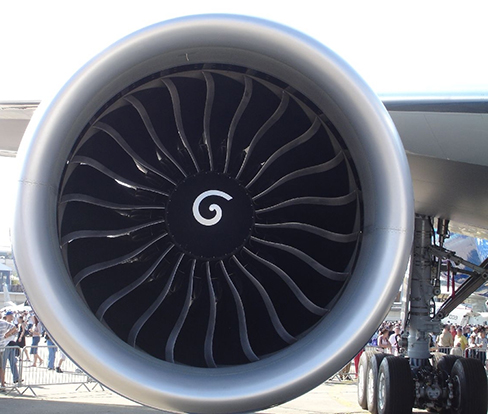Figure 10.9 (credit: “Bubinator”/ Wikimedia Commons)

### Example

#### Wind Turbine

A wind turbine ((Figure)) in a wind farm is being shut down for maintenance. It takes 30 s for the turbine to go from its operating angular velocity to a complete stop in which the angular velocity function is $\omega (t)=[{(t{\text{s}}^{-1}-30.0)}^{2}\text{/}100.0]\text{rad}\text{/}\text{s}$. If the turbine is rotating counterclockwise looking into the page, (a) what are the directions of the angular velocity and acceleration vectors? (b) What is the average angular acceleration? (c) What is the instantaneous angular acceleration at $t=0.0,15.0,30.0\,\text{s}?$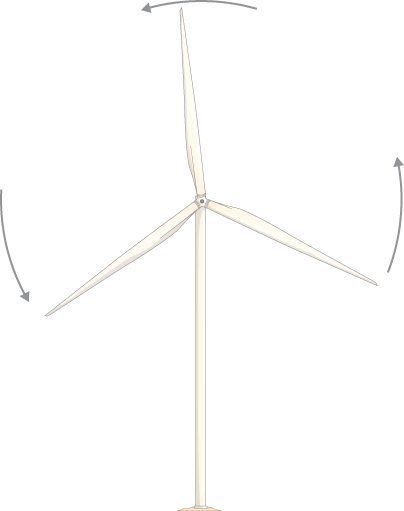Figure 10.10 A wind turbine that is rotating counterclockwise, as seen head on.

#### Strategy

1. We are given the rotational sense of the turbine, which is counterclockwise in the plane of the page. Using the right hand rule ((Figure)), we can establish the directions of the angular velocity and acceleration vectors.
2. We calculate the initial and final angular velocities to get the average angular acceleration. We establish the sign of the angular acceleration from the results in (a).
3. We are given the functional form of the angular velocity, so we can find the functional form of the angular acceleration function by taking its derivative with respect to time.

#### Solution

1. Since the turbine is rotating counterclockwise, angular velocity $\overset{\to }{\omega }$ points out of the page. But since the angular velocity is decreasing, the angular acceleration $\overset{\to }{\alpha }$ points into the page, in the opposite sense to the angular velocity.
2. The initial angular velocity of the turbine, setting $t=0,\,\text{is}\,\omega =9.0\,\text{rad}\text{/}\text{s}$. The final angular velocity is zero, so the average angular acceleration is
$\overset{–}{\alpha }=\frac{\text{Δ}\omega }{\text{Δ}t}=\frac{\omega -{\omega }_{0}}{t-{t}_{0}}=\frac{0-9.0\,\text{rad/s}}{30.0-0\,\text{s}}=-0.3\,\text{rad}\text{/}{\text{s}}^{2}.$
3. Taking the derivative of the angular velocity with respect to time gives $\alpha =\frac{d\omega }{dt}=(t-30.0)\text{/}50.0\,\text{rad}\text{/}{\text{s}}^{2}$
$\alpha (0.0\,\text{s})=-0.6\,\text{rad}\text{/}{\text{s}}^{2},\alpha (15.0\,\text{s})=-0.3\,\text{rad}\text{/}{\text{s}}^{2},\,\text{and}\,\alpha (30.0\,\text{s})=0\,\text{rad/s}.$

#### Significance

We found from the calculations in (a) and (b) that the angular acceleration $\alpha$ and the average angular acceleration $\overset{–}{\alpha }$ are negative. The turbine has an angular acceleration in the opposite sense to its angular velocity.

We now have a basic vocabulary for discussing fixed-axis rotational kinematics and relationships between rotational variables. We discuss more definitions and connections in the next section.

### Summary

• The angular position $\theta$ of a rotating body is the angle the body has rotated through in a fixed coordinate system, which serves as a frame of reference.
• The angular velocity of a rotating body about a fixed axis is defined as $\omega (\text{rad}\text{/}\text{s})$, the rotational rate of the body in radians per second. The instantaneous angular velocity of a rotating body $\omega =\underset{\text{Δ}t\to 0}{\text{lim}}\frac{\text{Δ}\omega }{\text{Δ}t}=\frac{d\theta }{dt}$ is the derivative with respect to time of the angular position $\theta$, found by taking the limit $\text{Δ}t\to 0$ in the average angular velocity $\overset{–}{\omega }=\frac{\text{Δ}\theta }{\text{Δ}t}$. The angular velocity relates ${v}_{\text{t}}$ to the tangential speed of a point on the rotating body through the relation ${v}_{\text{t}}=r\omega$, where r is the radius to the point and ${v}_{\text{t}}$ is the tangential speed at the given point.
• The angular velocity $\overset{\to }{\omega }$ is found using the right-hand rule. If the fingers curl in the direction of rotation about a fixed axis, the thumb points in the direction of $\overset{\to }{\omega }$ (see (Figure)).
• If the system’s angular velocity is not constant, then the system has an angular acceleration. The average angular acceleration over a given time interval is the change in angular velocity over this time interval, $\overset{–}{\alpha }=\frac{\text{Δ}\omega }{\text{Δ}t}$. The instantaneous angular acceleration is the time derivative of angular velocity, $\alpha =\underset{\text{Δ}t\to 0}{\text{lim}}\frac{\text{Δ}\omega }{\text{Δ}t}=\frac{d\omega }{dt}$. The angular acceleration $\overset{\to }{\alpha }$ is found by locating the angular velocity. If a rotation rate of a rotating body is decreasing, the angular acceleration is in the opposite direction to $\overset{\to }{\omega }$. If the rotation rate is increasing, the angular acceleration is in the same direction as $\overset{\to }{\omega }$.
• The tangential acceleration of a point at a radius from the axis of rotation is the angular acceleration times the radius to the point.

### Conceptual Questions

A clock is mounted on the wall. As you look at it, what is the direction of the angular velocity vector of the second hand?

What is the value of the angular acceleration of the second hand of the clock on the wall?

A baseball bat is swung. Do all points on the bat have the same angular velocity? The same tangential speed?

The blades of a blender on a counter are rotating clockwise as you look into it from the top. If the blender is put to a greater speed what direction is the angular acceleration of the blades?

### Problems

Calculate the angular velocity of Earth.

A track star runs a 400-m race on a 400-m circular track in 45 s. What is his angular velocity assuming a constant speed?

A wheel rotates at a constant rate of $2.0\,×\,{10}^{3}\,\text{rev}\text{/}\text{min}\,$. (a) What is its angular velocity in radians per second? (b) Through what angle does it turn in 10 s? Express the solution in radians and degrees.

A particle moves 3.0 m along a circle of radius 1.5 m. (a) Through what angle does it rotate? (b) If the particle makes this trip in 1.0 s at a constant speed, what is its angular velocity? (c) What is its acceleration?

A compact disc rotates at 500 rev/min. If the diameter of the disc is 120 mm, (a) what is the tangential speed of a point at the edge of the disc? (b) At a point halfway to the center of the disc?

Unreasonable results. The propeller of an aircraft is spinning at 10 rev/s when the pilot shuts off the engine. The propeller reduces its angular velocity at a constant $2.0\,\text{rad}\text{/}{\text{s}}^{2}$ for a time period of 40 s. What is the rotation rate of the propeller in 40 s? Is this a reasonable situation?

A gyroscope slows from an initial rate of 32.0 rad/s at a rate of $0.700{\,\text{rad/s}}^{2}$. How long does it take to come to rest?

On takeoff, the propellers on a UAV (unmanned aerial vehicle) increase their angular velocity from rest at a rate of $\omega =(25.0t)\,\text{rad}\text{/}\text{s}$ for 3.0 s. (a) What is the instantaneous angular velocity of the propellers at $t=2.0\,\text{s}$? (b) What is the angular acceleration?

The angular position of a rod varies as $20.0{t}^{2}$ radians from time $t=0$. The rod has two beads on it as shown in the following figure, one at 10 cm from the rotation axis and the other at 20 cm from the rotation axis. (a) What is the instantaneous angular velocity of the rod at $t=5\,\text{s}?$ (b) What is the angular acceleration of the rod? (c) What are the tangential speeds of the beads at $t=5\,\text{s}?$ (d) What are the tangential accelerations of the beads at $t=5\,\text{s}?$ (e) What are the centripetal accelerations of the beads at $t=5\,\text{s}?$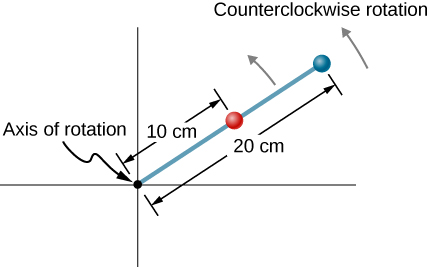### Glossary

angular acceleration
time rate of change of angular velocity
angular position
angle a body has rotated through in a fixed coordinate system
angular velocity
time rate of change of angular position
instantaneous angular acceleration
derivative of angular velocity with respect to time
instantaneous angular velocity
derivative of angular position with respect to time